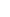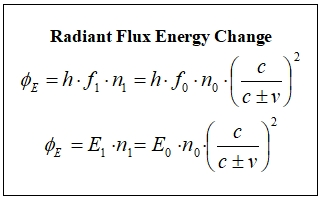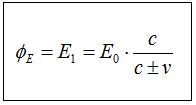34. RADIANT FLUX SHIFTThe definition of radiation is the emission or transfer of energy in the form of electromagnetic waves or particles. Radiant Flux is the radiant energy of electromagnetic radiation in a unit of time. Radiant Flux is not only about visible light; it includes all electromagnetic spectrum. Im limiting the topic with light sources that do photon radiation individually and randomly such as the sun or a lamp in order not to go too deep on the topic. Photon radiation occurs as the emission of electromagnetic waves in energy packs that are independent of each other.Our topic is about the effect of (c+v) (c-v) mathematics on Radiant Flux in electromagnetic waves. I dont think Alpha and Beta radiations that are particle radiations carrying mass are included in the scope of this topic. It is a remote possibility for these particles to behave in line with (c+v) (c-v) mathematics because they have masses.Radiant Flux is a measure of the number of photons are emitted in a unit of time when it is examined in terms of the source that emits the signal. When it is examined in terms of the arrival target of the signal, on the other hand, it is a measure of the number of photons are received. The energy that photons carry is also in this formation as a secondary factor that determines Radiant Flux. Consequently, Radiant Flux Shift is an effect that the change in the number of photons and the change in the energy of photons create together in the electromagnetic interaction between objects that are in motion relative to each other and it is a topic that is directly related to (c+v) (c-v) mathematics.The change in Radiant Flux of the source can occur only as a result of changes in the physical conditions at the source. Events like solar flares or change in the voltage of a lamp can change Radiant Flux of the source in its nature. We will discuss Radiant Flux here in terms of arrival target.We saw in the part Energy of a Photon that the amount of light energy that a reference system that is in motion relative to a light source increases or decreases depending on the direction of the movement. This change in the light energy does not only occur because of changing of wavelength (and therefore its energy); there is also an increase or decrease in the number of photos received, as well.In order to cover how Radiant Flux Shift occurs, we can make use of our Byte Shift example. Assuming that each alphabetical letter is represented by a photon in the message HELLO WORLD immediately gives us the necessary information.We saw that the length of the message that comes to the planes and the mountain station is different for each of the three reference systems. Since the INCOMING signal speed doesnt change for the target reference system, all three reference systems receive different amounts of photons in the same duration. As a result of this, Radiant Flux for all three reference systems is different. Assume that the signal tower emits n0 number of photons every second for each of the three arrival targets. Accordingly, when the mountain station receives n0 photons, the plane that is moving away from the towerreceives photons every second and the plane that is approaching the tower receivesphotons every second.Lets benefit from the frequency in order to find the change in the energy. The change in the n value that shows the number of photons that are emitted or received every second and the frequency change are so similar.Lets benefit from the frequency in order to find the change in the energy. The change in the n value that shows the number of photons that are emitted or received every second and the frequency change are so similar.Given that n0 photons set out towards the target every second and n1 of them will reach the target every second, if we multiply the sides of the equation with each other, we find the total effect that n1 photons that are at f1 frequency creates at the arrival target, i.e. the first equation of Radiant Flux. This equation shows the general principle in terms of (c+v) (c-v) mathematics; both frequency change (and therefore the wavelength change of the photon) and the change in the number of photons are included together in the equation.We obtain the energy change in Radiant Flux by multiplying the equation by Plancks constant.Of course, in order to use this equation, we need to know the number of the photon. But it has no importance for us in terms of our topic. The main purpose here was to see how (c+v) (c-v) mathematics was involved in this event. It is possible to eliminate the number of photons, anyway. We can simplify Radiant Flux Shift equation above as can be seen below. Since, if we put the values in their places in the previous equation, we get the general equation:The equationthat indicates the amount of Radiant Flux Shift shows the amount of change in the radiant flux, which a reference system that is in motion relative to the source, will be exposed to.We can summarize the formation of Radiant Flux Shift as follows:  Radiant Flux Shift occurs at the source just like wavelength change and its effect shows up in the target. The fact that a reference system is in motion relative to a light source increases or decreases the density of the light that comes from the source to the reference system itself. In other words, it changes the density of the photons that come to it. This density change in the number of photons occurs during the emission of the light. For the photons that set out to show their effects, they need to cover the distance between the source and the arrival target. Therefore, Radiant Flux Shift is not an effect that occurs at that exact moment on the target depending on the change in the speed.It will be easier to talk about this by giving an example. Assume that we set out at half a light-year speed towards another solar system that is at distance of four light-years. For the spaceship, the change in the radiant flux of the light that comes from the star will start exactly four years after we set out, when the half of the distance is covered. Whenever the spaceship starts to see the wavelength change of the lights coming from the star, Radiant Flux of the lights that reach the spaceship from the star will then change.